Background
If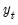is the dependent (autoregressive) variable,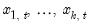are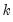distributed-lag explanatory variables, and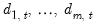are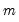exogenous, potentially deterministic variables, the Intertemporal Dynamics (ITD) representation of an ARDL(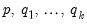) model is given by: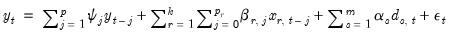(29.1)
where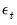are the innovations, and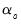,, and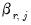are the coefficients associated with the exogenous variables,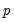lags of, and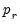lags of thedistributed lag regressors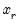, respectively.
Let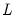be the usual lag operator and define the lag polynomials: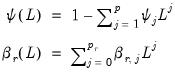(29.2)
Substituting into Equation (29.1) yields: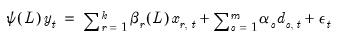(29.3)
Noting that any series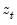may be written as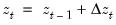and performing a Beveridge-Nelson decomposition on both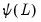and the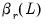in Equation (29.3) produces the Conditional Error Correction (CEC) representation of the ARDL,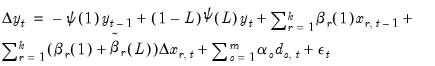(29.4)
which, with a bit of manipulation, may be rewritten as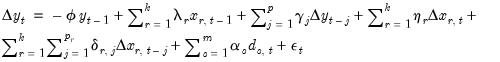(29.5)
where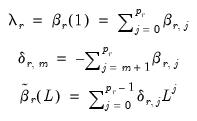(29.6)
and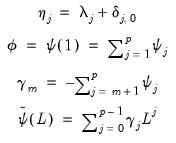(29.7)
Since CEC Equation (29.5) and Equation (29.8) are derived from ITD Equation (29.1), there is an obvious one-to-one correspondence between the two. As with the vector error correction (VEC) form of a VAR, the CEC form offers easy identification of a cointegrating relationship between the dependent variable and the explanatory variables in the ARDL. We discuss this parallel in greater depth in “Relationship to Vector Error Correction (VEC) Models”.
Rearrange terms, we may re-write Equation (29.8) as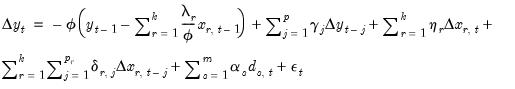(29.8)
If we define the equilibrium error correction term,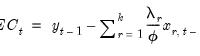(29.9)
then Equation (29.8) may be written in Error Correction (EC) form: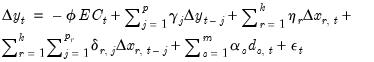(29.10)
where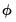is the error correction parameter, and the long-term equilibrium parameters for the explanatory variables are given by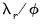, for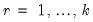.
Conveniently, the coefficients in both the ITD and the CEC representations of the ARDL model may be estimated via least squares.
Relationship to Vector Error Correction (VEC) Models
Assuming the same lag across the distributed-lag regressors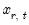and that the deterministics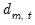consist of a simple constant and linear trend, Pesaran (2001) demonstrates that the ARDL CEC representation in Equation (29.8) is in fact the CEC of the VAR() model: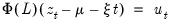(29.11)
where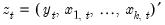is a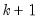vector of endogenous variables,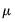and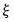are thevectors of intercept and trend coefficients, respectively, and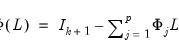(29.12)
is thematrix lag polynomial.
Invoking the BN decomposition on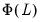and with following some rearrangement, the CEC representation of this VEC may be written as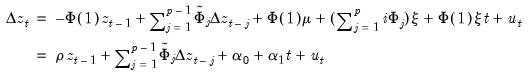(29.13)
where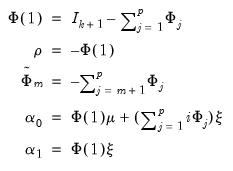(29.14)
which is equivalent to Equation (29.5).
Nonlinear (asymmetric) ARDL
The classical ARDL framework assumes that the long-run relationship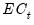is a symmetric linear combination of regressors. While this is a natural starting assumption, it does not match the behavioral finance and economics literature approach to modeling nonlinearity and asymmetry (Kahneman, Tversky, and Shiller, 1979). In response, Shin (2014) proposes a nonlinear ARDL (NARDL) framework in which short-run and long-run nonlinearities are modeled as positive and negative partial sum decompositions of the explanatory variables.
Consider the partial sum decomposition of a variablearound a threshold of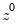as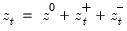where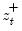and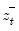are the partial sum processes of positive and negative changes in, respectively: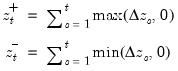(29.15)
The ITD representation of a NARDL() model is given by: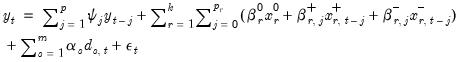(29.16)
where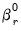are coefficients for the initial conditions, and where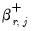and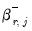are coefficients associated with the asymmetric distributed-lag variables.
We may an obtain a CEC representation of the ITD NARDL model,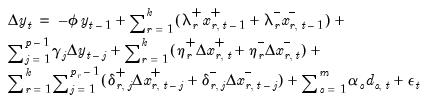(29.17)
where the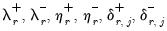are asymmetric analogues of the coefficients in Equation (29.6).
We may rearrange terms so that Equation (29.17) becomes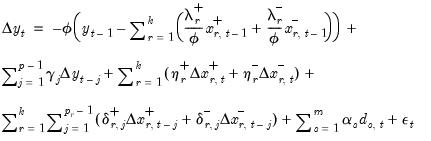(29.18)
Then, define the asymmetric equilibrium error correction term,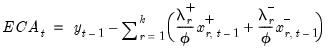(29.19)
so that the CEC Equation (29.18) may be written in EC form: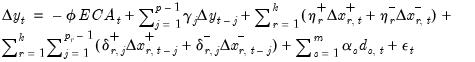(29.20)
whereis the error correction parameter, the long-term equilibrium parameters for the explanatory variables are given by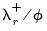and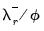for. The short-run parameters for the explanatory variables are given by the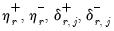.
Notice that because the CEC representation decomposes the effect of the distribution lag variables into short and long-run components, it allows for asymmetries in various combinations of short and long-run dynamics. This flexibility does not exist in the ITD representation.
As with their symmetric counterparts, NARDL models may be estimated via least squares. This result is appealing since nonlinear models often require iterative estimation routines. Furthermore, bounds testing procedures ( “Bounds Test View”) remain valid and require no meaningful adjustments.